# shapviz

## Introduction

SHAP (SHapley Additive exPlanations, see Lundberg and Lee (2017)) is an ingenious way to study black box models. SHAP values decompose - as fair as possible - predictions into additive feature contributions. Crunching SHAP values requires clever algorithms by clever people. Analyzing them, however, is super easy with the right visualizations. The “shapviz” package offers the latter:

• `sv_dependence()`: Dependence plots to study feature effects and interactions.
• `sv_importance()`: Importance plots (bar plots and/or beeswarm plots) to study variable importance.
• `sv_interaction()`: Interaction plots.
• `sv_waterfall()`: Waterfall plots to study single predictions.
• `sv_force()`: Force plots as an alternative to waterfall plots.

These plots require a “shapviz” object, which is built from two things only:

1. `S`: Matrix of SHAP values
2. `X`: Dataset that includes the corresponding feature values

Optionally, a `baseline` can be passed to represent an average prediction on the scale of the SHAP values. Also a 3D array of SHAP interaction values can be passed as `S_inter`.

A key feature of “shapviz” is that `X` is used for visualization only. Thus it is perfectly fine to use factor variables, even if the underlying model would not accept these. Additionally, in order to improve visualization, it can sometimes make sense to clip gross outliers, take logarithms for certain columns, or replace missing values by some explicit value.

To further simplify the use of “shapviz”, we added direct connectors to these R packages:

For XGBoost, LightGBM, and H2O, the SHAP values are directly calculated from the fitted model.

`CatBoost` is not included, but see Section “Any other package” how to use its SHAP calculation backend with “shapviz”.

## Installation

``````# From CRAN
install.packages("shapviz")

# Or the newest version from GitHub:
# install.packages("devtools")
devtools::install_github("mayer79/shapviz")``````

## Example: Diamond prices

### Fit model

We start by fitting an XGBoost model to predict diamond prices based on the four “C” features.

``````library(shapviz)
library(ggplot2)
library(xgboost)

set.seed(3653)

# Turn ordinal factors into normal ones
ord <- c("clarity", "cut", "color")
diamonds[, ord] <- lapply(diamonds[, ord], factor, ordered = FALSE)

# Fit XGBoost model
x <- c("carat", "clarity", "cut", "color")
dtrain <- xgb.DMatrix(data.matrix(diamonds[x]), label = diamonds\$price)

fit <- xgb.train(
params = list(learning_rate = 0.1, objective = "reg:squarederror"),
data = dtrain,
nrounds = 65L
)``````

### Create “shapviz” object

One line of code creates a “shapviz” object. It contains SHAP values and feature values for the set of observations we are interested in. Note again that `X` is solely used as explanation dataset, not for calculating SHAP values.

In this example we construct the “shapviz” object directly from the fitted XGBoost model. Thus we also need to pass a corresponding prediction dataset `X_pred` used for calculating SHAP values by XGBoost.

``````# Pick explanation data
dia_small <- diamonds[sample(nrow(diamonds), 2000L), ]

# We also pass feature data X with originally encoded values
shp <- shapviz(fit, X_pred = data.matrix(dia_small[x]), X = dia_small)``````

Note: If `X_pred` would contain one-hot-encoded dummy variables, their SHAP values (and also SHAP interaction values) could be collapsed by the `collapse` argument of `shapviz()`.

### Decompose single prediction

The main idea behind SHAP values is to decompose, in a fair way, a prediction into additive contributions of each feature. Typical visualizations include waterfall plots and force plots:

``````sv_waterfall(shp, row_id = 1L) +
theme(axis.text = element_text(size = 11))``````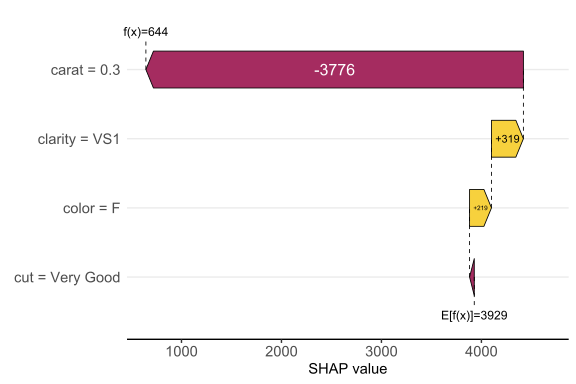Works pretty sweet, and factor input is respected!

Alternatively, we can study a force plot:

``sv_force(shp, row_id = 1L)``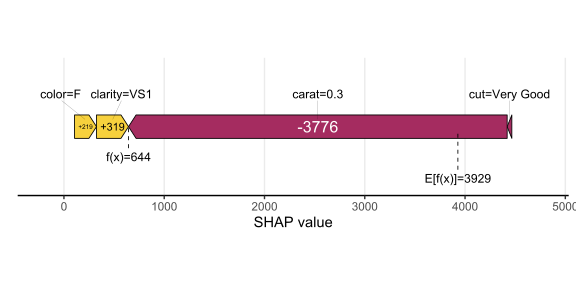### SHAP importance

Studying SHAP decompositions of many observations allows to gain an impression on variable importance. As simple descriptive measure, the mean absolute SHAP value of each feature is considered. These values can be plotted as a simple bar plot, or, to add information on the sign of the feature effects, as a beeswarm plot sorted by the mean absolute SHAP values. Such beeswarm plots are often called “summary plots”.

``````# A bar plot of mean absolute SHAP values
sv_importance(shp)``````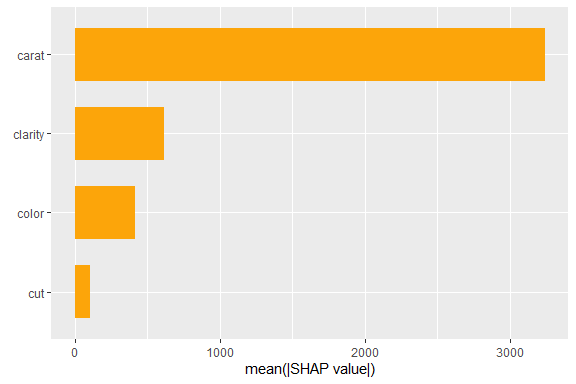``````
# A beeswarm plot
sv_importance(shp, kind = "beeswarm")``````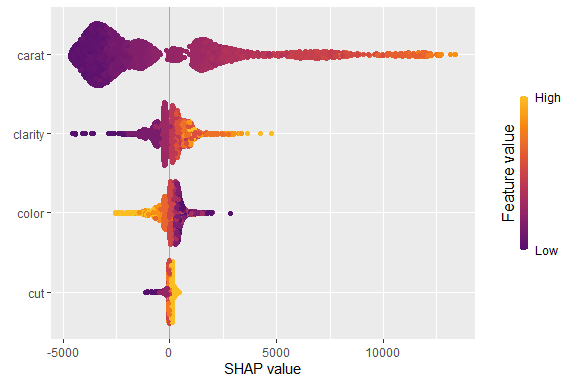``````
# Or both!
sv_importance(shp, kind = "both", show_numbers = TRUE, bee_width = 0.2)``````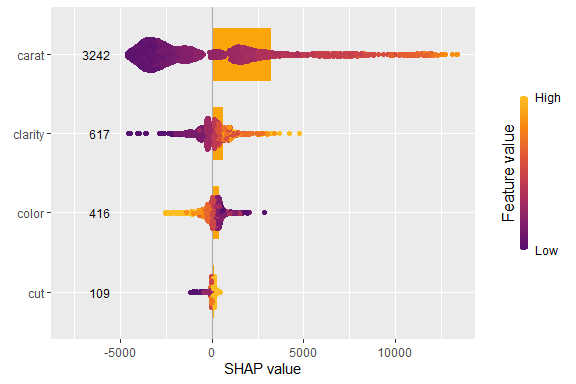### SHAP dependence plots

A SHAP beeswarm importance plot gives first hints on whether high feature values tend to high or low predictions. This impression can be substantiated by studying simple scatterplots of SHAP values of a feature against its feature values.

On the color axis, the feature with (heuristically) strongest interaction is shown by default. Use `color_var` to use another feature (or `NULL` for no coloring).

``sv_dependence(shp, v = "color")``